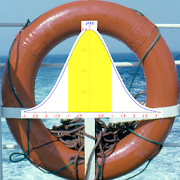# SOS-StochasticsEveryone
This programme offers modules to the following stochastics themes:
Binomial Distribution
Normal Distribution
Hypotheses Tests
Confidence Intervals
Probability Tree
In the module "Binomial Distribution" you can calculate the probabilities of a Bernoulli-chain and visualize the results in a graphical representation. It is possible to mark a region corresponding to a sum probability. A normal distribution curve or an approximation by a Poisson distribution can be shown as a reference.
The part "Normal Distribution" uses the probability mass function as an approximation to the binomial distribution for a large sample size. Here, too, sum probabilities can be calculated and emphasized in the graphical representation. Optionally, the graph of the cumulative distribution function can be shown.
The module "Hypotheses Tests" contains four different kinds of representations. On the basis of a binomial or normal distribution the risk of the 1st or 2nd kind for one- or two-sided tests can be calculated and visualized. In order to judge the test situation there is an option to show the graph of the power function or the OC-function.
The module "Confidence Intervals" lets you represent graphically the relative frequency of up to 20 characters in a bar chart. For each relative frequency the corresponding confidence interval is calculated and can be shown. The level of confidence can be specified. One important application is the interpretation of surveys before elections.
The module "Probability Tree" lets you define up to 5 levels of random trials with two exits. On each level the success probability can be specified independently. From these settings the corresponding probability tree is constructed. All occuring probabilities can be shown.
Collapse

Review Policy

## What's New

Changed: Font sizes in some graphical representations.
Collapse

Eligible for Family LibraryEligible if bought after 7/2/2016. Learn More
Updated
February 12, 2019
Size
1.4M
Installs
50+
Current Version
1.1
Requires Android
5.1 and up
Content Rating
Everyone
Permissions
Offered By
carolus7490
Developer
Meisenweg 4 D-25436 Uetersen